﻿ C : Insert new node at any position in a doubly linked list

# C Exercises: Insert new node at any position in a doubly linked list

## C Doubly Linked List : Exercise-5 with Solution

Write a program in C to insert a new node at any position in a doubly linked list.

Pictorial Presentation: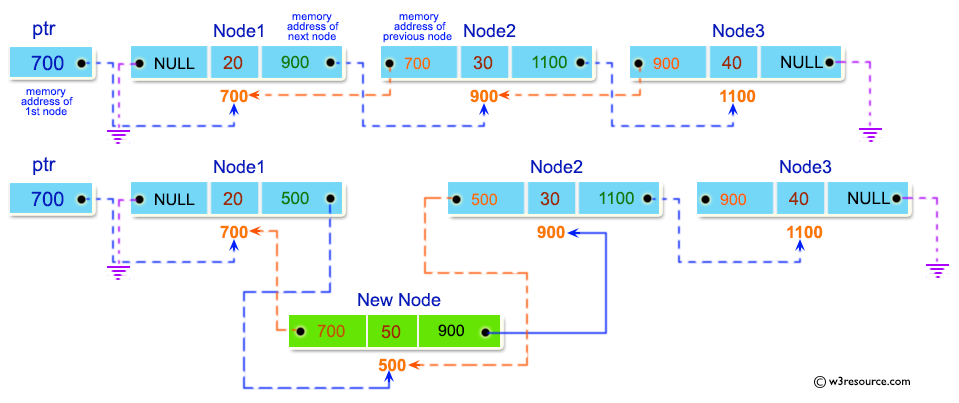Sample Solution:

C Code:

``````#include <stdio.h>
#include <stdlib.h>

struct node {
int num;
struct node * preptr;
struct node * nextptr;
}*stnode, *ennode;

void DlListcreation(int n);
void DlLinsertNodeAtBeginning(int num);
void DlLinsertNodeAtEnd(int num);
void DlLinsertNodeAtAny(int num, int pos);
void displayDlList(int a);

int main()
{
int n,num1,a,insPlc;
stnode = NULL;
ennode = NULL;
printf("\n\n Doubly Linked List : Insert new node at any position in a doubly linked list :\n");
printf("-----------------------------------------------------------------------------------\n");
printf(" Input the number of nodes : ");
scanf("%d", &n);
DlListcreation(n);
a=1;
displayDlList(a);
printf(" Input the position ( 1 to %d ) to insert a new node : ",n+1);
scanf("%d", &insPlc);
printf(" Input data for the position %d : ", insPlc);
scanf("%d", &num1);
DlLinsertNodeAtAny(num1,insPlc);
a=2;
displayDlList(a);
return 0;
}

void DlListcreation(int n)
{
int i, num;
struct node *fnNode;

if(n >= 1)
{
stnode = (struct node *)malloc(sizeof(struct node));

if(stnode != NULL)
{
printf(" Input data for node 1 : "); // assigning data in the first node
scanf("%d", &num);

stnode->num = num;
stnode->preptr = NULL;
stnode->nextptr = NULL;
ennode = stnode;
for(i=2; i<=n; i++)
{
fnNode = (struct node *)malloc(sizeof(struct node));
if(fnNode != NULL)
{
printf(" Input data for node %d : ", i);
scanf("%d", &num);
fnNode->num = num;
fnNode->preptr = ennode;    // new node is linking with the previous node
fnNode->nextptr = NULL;     // set next address of fnnode is NULL
ennode->nextptr = fnNode;   // previous node is linking with the new node
ennode = fnNode;            // assign new node as last node
}
else
{
printf(" Memory can not be allocated.");
break;
}
}
}
else
{
printf(" Memory can not be allocated.");
}
}
}

void DlLinsertNodeAtAny(int num, int pos)
{
int i;
struct node * newnode, *tmp;
if(ennode == NULL)
{
printf(" No data found in the list!\n");
}
else
{
tmp = stnode;
i=1;
while(i<pos-1 && tmp!=NULL)
{
tmp = tmp->nextptr;
i++;
}
if(pos == 1)
{
DlLinsertNodeAtBeginning(num);
}
else if(tmp == ennode)
{
DlLinsertNodeAtEnd(num);
}
else if(tmp!=NULL)
{
newnode = (struct node *)malloc(sizeof(struct node));
newnode->num = num;
newnode->nextptr = tmp->nextptr;
// previous address of new node is linking with the tmp node
newnode->preptr = tmp;
if(tmp->nextptr != NULL)
{
tmp->nextptr->preptr = newnode; // n+1th node is linking with new node
}
tmp->nextptr = newnode; // n-1th node is linking with new node
}
else
{
printf(" The position you entered, is invalid.\n");
}
}
}
void DlLinsertNodeAtBeginning(int num)
{
struct node * newnode;
if(stnode == NULL)
{
printf(" No data found in the list!\n");
}
else
{
newnode = (struct node *)malloc(sizeof(struct node));
newnode->num = num;
newnode->nextptr = stnode;  // next address of new node is linking with starting node
newnode->preptr = NULL;     // set previous address field of new node is NULL
stnode->preptr = newnode;   // previous address of starting node is linking with new node
stnode = newnode;           // set the new node as starting node
}
}

void DlLinsertNodeAtEnd(int num)
{
struct node * newnode;

if(ennode == NULL)
{
printf(" No data found in the list!\n");
}
else
{
newnode = (struct node *)malloc(sizeof(struct node));
newnode->num = num;
newnode->nextptr = NULL;        // set next address field of new node  is NULL
newnode->preptr = ennode;       // previous address of new node is linking with ending node
ennode->nextptr = newnode;      // next address of ending node is linking with new node
ennode = newnode;               // set the new node as ending node
}
}

void displayDlList(int m)
{
struct node * tmp;
int n = 1;
if(stnode == NULL)
{
printf(" No data found in the List yet.");
}
else
{
tmp = stnode;
if (m==1)
{
printf("\n Data entered in the list are :\n");
}
else
{
printf("\n After insertion the new list are :\n");
}
while(tmp != NULL)
{
printf(" node %d : %d\n", n, tmp->num);
n++;
tmp = tmp->nextptr; // current pointer moves to the next node
}
}
}
```
```

Sample Output:

``` Doubly Linked List : Insert new node at any position in a doubly linked list :
-----------------------------------------------------------------------------------
Input the number of nodes : 3
Input data for node 1 : 2
Input data for node 2 : 5
Input data for node 3 : 8

Data entered in the list are :
node 1 : 2
node 2 : 5
node 3 : 8
Input the position ( 1 to 4 ) to insert a new node : 4
Input data for the position 4 : 9

After insertion the new list are :
node 1 : 2
node 2 : 5
node 3 : 8
node 4 : 9
```

Flowchart: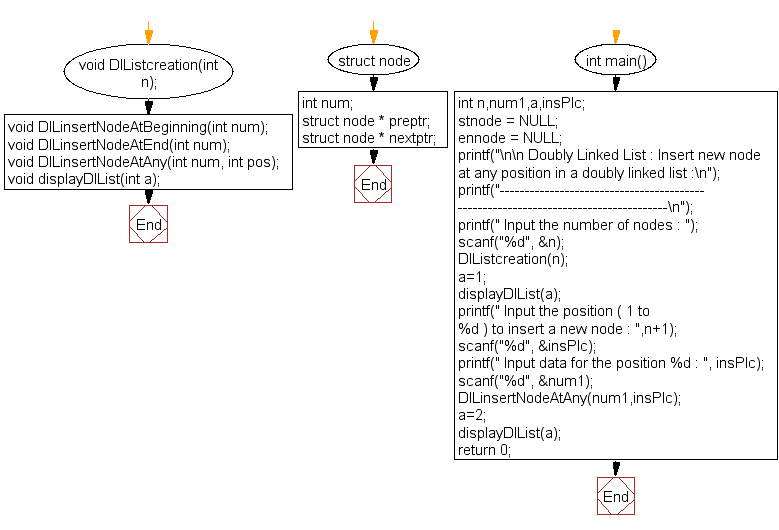DlListcreation() :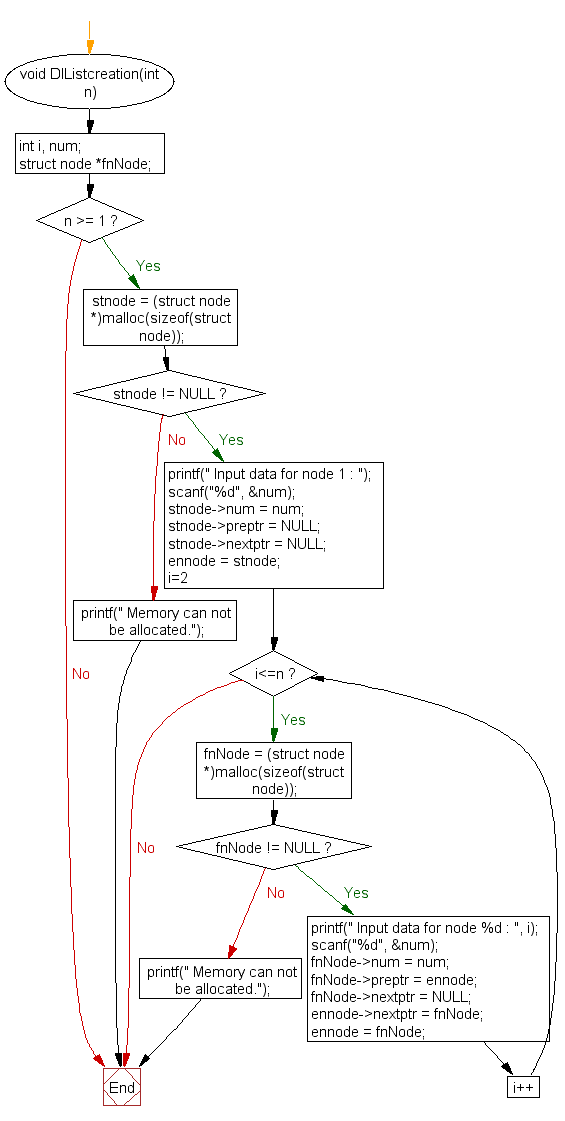DlLinsertNodeAtAny() :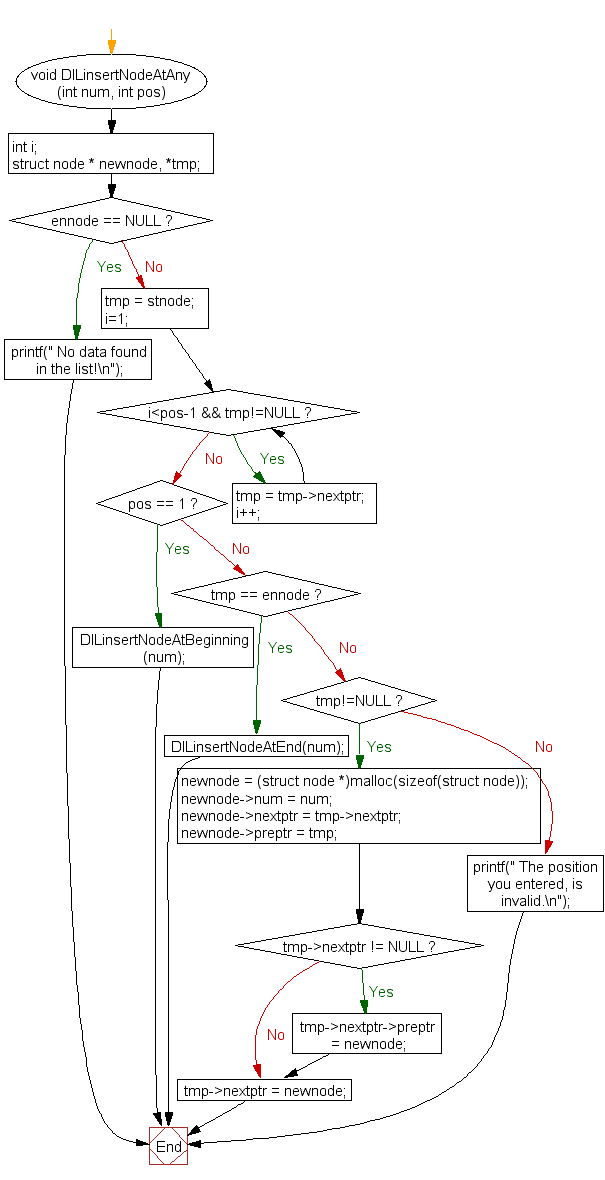DlLinsertNodeAtBeginning() :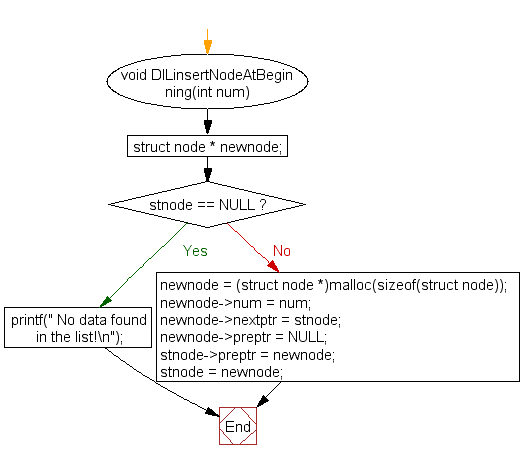DlLinsertNodeAtEnd() :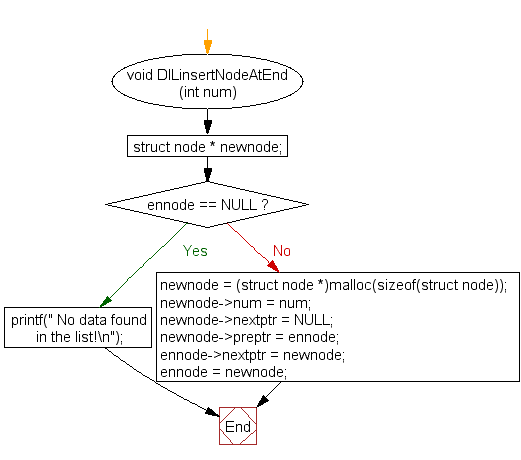displayDlList() :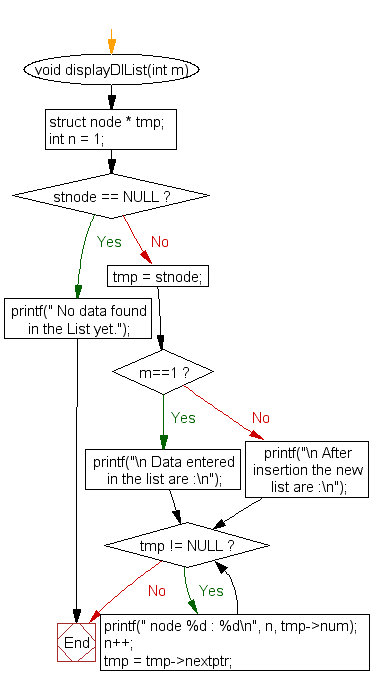C Programming Code Editor:

What is the difficulty level of this exercise?

Test your Programming skills with w3resource's quiz.

﻿